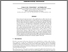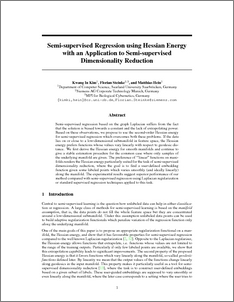# Semi-supervised regression using Hessian energy with an application to semi-supervised dimensionality reduction

Kim, Kwang In and Steinke, Florian and Hein, Matthias (2010) Semi-supervised regression using Hessian energy with an application to semi-supervised dimensionality reduction. In: Advances in Neural Information Processing Systems (NIPS). MPI for Biological Cybernetics, Germany, pp. 979-987.Preview
PDF (\\lancs\homes\60\zhangy3\My Desktop\PURE PDF\Semi)
Semi.pdf - Submitted Version

## Abstract

Semi-supervised regression based on the graph Laplacian suffers from the fact that the solution is biased towards a constant and the lack of extrapolating power. Based on these observations, we propose to use the second-order Hessian energy for semi-supervised regression which overcomes both these problems. If the data lies on or close to a low-dimensional submanifold in feature space, the Hessian energy prefers functions whose values vary linearly with respect to geodesic distance. We first derive the Hessian energy for smooth manifolds and continue to give a stable estimation procedure for the common case where only samples of the underlying manifold are given. The preference of ‘’linear” functions on manifolds renders the Hessian energy particularly suited for the task of semi-supervised dimensionality reduction, where the goal is to find a user-defined embedding function given some labeled points which varies smoothly (and ideally linearly) along the manifold. The experimental results suggest superior performance of our method compared with semi-supervised regression using Laplacian regularization or standard supervised regression techniques applied to this task.

Item Type:
Contribution in Book/Report/Proceedings
ID Code:
69844
Deposited By:
Deposited On:
03 Jul 2014 10:36
Refereed?:
Yes
Published?:
Published